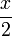# User talk:Emanuele

(Difference between revisions)
Emanuele (Talk | contribs)
(New page: testing math equations $\frac{x}{2}$ $x^2+3x+5$)

## Revision as of 22:04, 14 January 2010

testing math equations$\frac{x}{2}$

x2 + 3x + 5